An algebraover a fieldsuch that for any elementsandthe equations,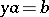are solvable in. An associative division algebra, considered as a ring, is a skew-field, its centreis a field, and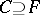. If, the division algebrais called a central division algebra. Finite-dimensional central associative division algebras overmay be identified, up to an isomorphism, with the elements of the Brauer group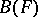of the field. Let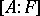denote the dimension ofover. If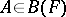and ifis the maximal subfield in(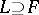), then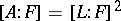. According to the Frobenius theorem, all associative finite-dimensional division algebras over the field of real numbersare exhausted byitself, the field of complex numbers, and the quaternion algebra. For this reason the groupis cyclic of order two. If the associativity requirement is dropped, there is yet another example of a division algebra over the field of real numbers: the Cayley–Dickson algebra. This algebra is alternative, and its dimension overis 8. Ifis a finite-dimensional (not necessarily associative) division algebra over, then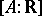has one of the values 1, 2, 4, or 8.